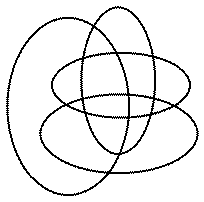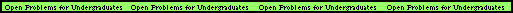# Venn Diagrams Open Problems

Index of Problems

### Venn diagrams with 6 triangles

RESEARCHER: Peter Hamburger
OFFICE: CoRE 442
Email:hamburge@dimacs.rutgers.edu
DESCRIPTION:The figure to the right is a Venn diagram with 4 closed curves. The curves in this figure are ellipses. It is a Venn diagram because each of the 2^4 regions created by the curves (including the exterior to all of them) is in the interior of a different subset of the 4 curves from the other regions. Thus each possible subset of the 4 curves has a unique region which is contained inside exactly that subset of curves. The exterior region is contained inside the empty subset of curves.

The largest Venn diagram that can be created with circles consists of 3 circles...you've probably seen it. Can you prove that no Venn diagram can be created with 4 circles? This is not too hard. The largest Venn diagram that can be created with ellipses has 5 ellipses, was discovered only recently, and was thought for a long time not to exist!

It is possible to create a Venn diagram with 5 triangles, but this is tricky. The open problem is this: is it possible to create a Venn diagram with 6 triangles?

For more information, you may wish to check out Simple Reducible Venn Diagrams on Five Curves and Hamiltonian Cycles, a postscript file.### Peter Winkler's Conjecture

RESEARCHER: Peter Hamburger
OFFICE: CoRE 442
Email:hamburge@dimacs.rutgers.edu
DESCRIPTION:A Venn diagram is called "simple" if curves cross when they meet (no tangencies) and if no 3 curves meet at a point. The Venn diagram in the previous problem is simple.

Winkler conjectured about 15 years ago that any simple Venn diagram could be extended to a larger simple Venn diagram with the addition of a single curve. The figure to the right shows how to add a curve to the Venn diagram above to obtain a larger simple Venn diagram. Winkler's conjecture is still open.

If you consider a Venn diagram to be a planar graph, where the vertices are the points of intersection of the curves and the edges are the arcs of the curves between vertices, then Winkler's conjecture amounts to showing that the dual of this graph is Hamiltonian. Can you see why this is the case? It isn't too hard to see. So, to prove Winkler's conjecture, you can prove that the dual graph to any simple Venn diagram is Hamiltonian.

For more information, you may wish to check out Simple Reducible Venn Diagrams on Five Curves and Hamiltonian Cycles, a postscript file.Back to main page